Author: Oscar Cronquist Article last updated on July 14, 2022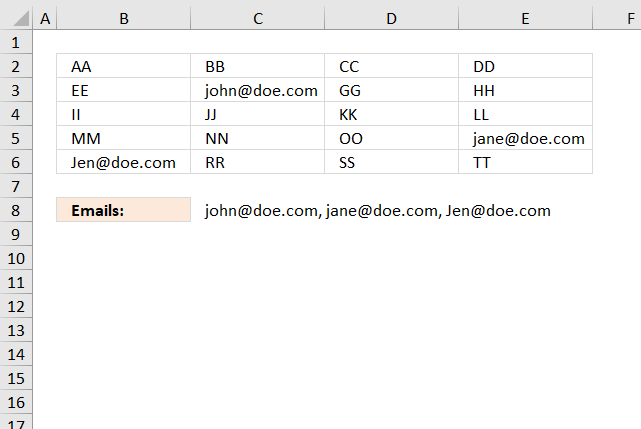Question: How to extract email addresses from this sheet?

It depends on how the emails are populated in your worksheet?

• Are they in a single cell each?
• Are there other text strings in the cell as well?

## Example 1,The following formula works if a cell contains only an email address, see image above. The TEXTJOIN function extracts all emails based on if character @ is found in the cell.

Array formula in cell C8:

=TEXTJOIN(", ", TRUE, IF(ISERROR(SEARCH("@", B2:E6)), "", B2:E6))

####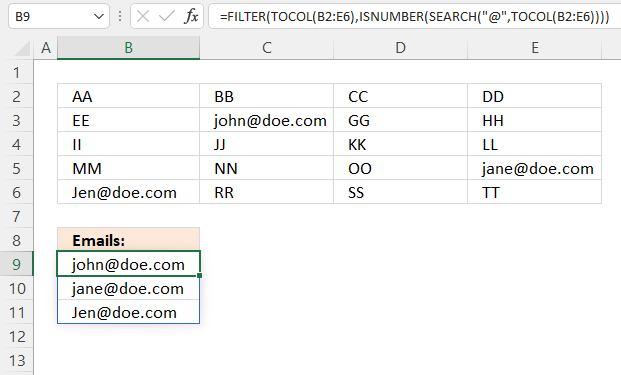Excel 365 formula in cell B9:

=FILTER(TOCOL(B2:E6),ISNUMBER(SEARCH("@",TOCOL(B2:E6))))

### Explaining formula

#### Step 1 - Rearrange array to a single column array

The TOCOL function rearranges values in 2D cell ranges to a single column.

Function syntax: TOCOL(array, [ignore], [scan_by_col])

TOCOL(B2:E6)

becomes

TOCOL({"AA", "BB", "CC", "DD"; "EE", "[email protected]", "GG", "HH"; "II", "JJ", "KK", "LL"; "MM", "NN", "OO", "[email protected]"; "[email protected]", "RR", "SS", "TT"})

and returns

{"AA"; "BB"; "CC"; "DD"; "EE"; "[email protected]"; "GG"; "HH"; "II"; "JJ"; "KK"; "LL"; "MM"; "NN"; "OO"; "[email protected]"; "[email protected]"; "RR"; "SS"; "TT"}

#### Step 2 - Search for character @

The SEARCH function returns the number of the character at which a specific character or text string is found reading left to right (not case-sensitive)

Function syntax: SEARCH(find_text,within_text, [start_num])

SEARCH("@",TOCOL(B2:E6))

becomes

SEARCH("@", {"AA"; "BB"; "CC"; "DD"; "EE"; "[email protected]"; "GG"; "HH"; "II"; "JJ"; "KK"; "LL"; "MM"; "NN"; "OO"; "[email protected]"; "[email protected]"; "RR"; "SS"; "TT"})

and returns

{#VALUE!; #VALUE!; #VALUE!; #VALUE!; #VALUE!; 5; #VALUE!; #VALUE!; #VALUE!; #VALUE!; #VALUE!; #VALUE!; #VALUE!; #VALUE!; #VALUE!; 5; 4; #VALUE!; #VALUE!; #VALUE!}

#### Step 3 - Look for numbers

The ISNUMBER function checks if a value is a number, returns TRUE or FALSE.

Function syntax: ISNUMBER(value)

ISNUMBER(SEARCH("@",TOCOL(B2:E6)))

becomes

ISNUMBER({#VALUE!; #VALUE!; #VALUE!; #VALUE!; #VALUE!; 5; #VALUE!; #VALUE!; #VALUE!; #VALUE!; #VALUE!; #VALUE!; #VALUE!; #VALUE!; #VALUE!; 5; 4; #VALUE!; #VALUE!; #VALUE!})

and returns

{FALSE; FALSE; FALSE; FALSE; FALSE; TRUE; FALSE; FALSE; FALSE; FALSE; FALSE; FALSE; FALSE; FALSE; FALSE; TRUE; TRUE; FALSE; FALSE; FALSE}

#### Step 4 - Filter values based on corresponding boolean values

The FILTER function extracts values/rows based on a condition or criteria.

Function syntax: FILTER(array, include, [if_empty])

FILTER(TOCOL(B2:E6),ISNUMBER(SEARCH("@",TOCOL(B2:E6))))

becomes

FILTER({"AA"; "BB"; "CC"; "DD"; "EE"; "[email protected]"; "GG"; "HH"; "II"; "JJ"; "KK"; "LL"; "MM"; "NN"; "OO"; "[email protected]"; "[email protected]"; "RR"; "SS"; "TT"}, {FALSE; FALSE; FALSE; FALSE; FALSE; TRUE; FALSE; FALSE; FALSE; FALSE; FALSE; FALSE; FALSE; FALSE; FALSE; TRUE; TRUE; FALSE; FALSE; FALSE})

and returns

## Example 2,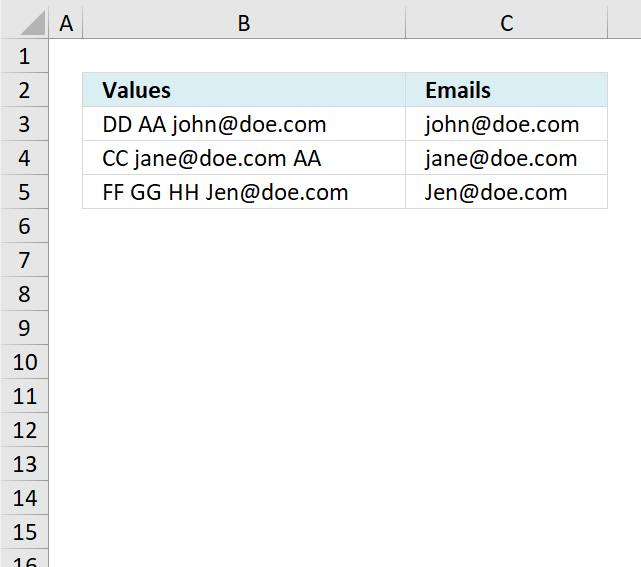The example above has multiple text strings in each cell separated by a blank, the formula is only capable of extracting one email address per cell and if the delimiting character is a blank (space). You can change the formula to use any delimiting character, however, only one delimiting character per formula.

Formula in cell C3:

=TRIM(MID(SUBSTITUTE(TRIM(B3), " ", REPT(" ", 200)), (LEN(LEFT(B3, SEARCH("@", B3)))-LEN(SUBSTITUTE(LEFT(B3, SEARCH("@", B3))," ", "")))*200+1, 200))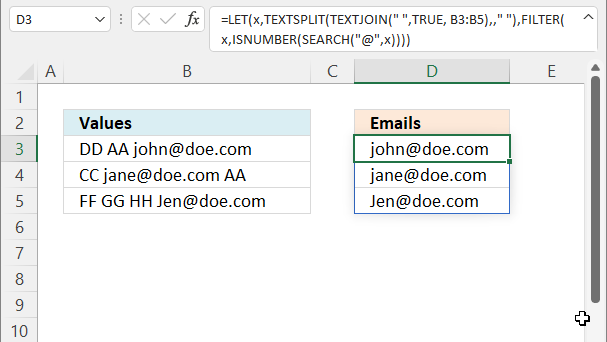Excel 365 formula in cell D3:

=LET(x,TEXTSPLIT(TEXTJOIN(" ",TRUE, B3:B5),," "),FILTER(x,ISNUMBER(SEARCH("@",x))))

### Explaining formula

The TEXTJOIN function has a limit of 32767 characters, the formula returns an error if you add more characters than that.

#### Step 1  - Merge strings in multiple cells

The TEXTJOIN function combines text strings from multiple cell ranges.

Function syntax: TEXTJOIN(delimiter, ignore_empty, text1, [text2], ...)

TEXTJOIN(" ",TRUE, B3:B5)

becomes

TEXTJOIN(" ",TRUE, {"DD AA [email protected]";"CC [email protected] AA";"FF GG HH [email protected]"})

and returns

"DD AA [email protected] CC [email protected] AA FF GG HH [email protected]"

#### Step 2  - Split strings based on a space character as a delimiting value

The TEXTSPLIT function splits a string into an array based on delimiting values.

Function syntax: TEXTSPLIT(Input_Text, col_delimiter, [row_delimiter], [Ignore_Empty])

TEXTSPLIT(TEXTJOIN(" ",TRUE, B3:B5),," ")

becomes

TEXTSPLIT("DD AA [email protected] CC [email protected] AA FF GG HH [email protected]",," ")

and returns

{"DD"; "AA"; "[email protected]"; "CC"; "[email protected]"; "AA"; "FF"; "GG"; "HH"; "[email protected]"}.

#### Step 3  - Search for a @ character

The SEARCH function returns the number of the character at which a specific character or text string is found reading left to right (not case-sensitive)

Function syntax: SEARCH(find_text,within_text, [start_num])

SEARCH("@",TEXTSPLIT(TEXTJOIN(" ",TRUE, B3:B5),," "))

becomes

SEARCH("@",{"DD"; "AA"; "[email protected]"; "CC"; "[email protected]"; "AA"; "FF"; "GG"; "HH"; "[email protected]"})

and returns

{#VALUE!; #VALUE!; 5; #VALUE!; 5; #VALUE!; #VALUE!; #VALUE!; #VALUE!; 4}.

#### Step 4  - Find numbers in array

The ISNUMBER function checks if a value is a number, returns TRUE or FALSE.

Function syntax: ISNUMBER(value)

ISNUMBER(SEARCH("@",TEXTSPLIT(TEXTJOIN(" ",TRUE, B3:B5),," ")))

becomes

ISNUMBER({#VALUE!; #VALUE!; 5; #VALUE!; 5; #VALUE!; #VALUE!; #VALUE!; #VALUE!; 4})

and returns

{FALSE; FALSE; TRUE; FALSE; TRUE; FALSE; FALSE; FALSE; FALSE; TRUE}.

#### Step 5  - Filter values based on correpsonding boolean array

The FILTER function extracts values/rows based on a condition or criteria.

Function syntax: FILTER(array, include, [if_empty])

FILTER(TEXTSPLIT(TEXTJOIN(" ",TRUE, B3:B5),," "),ISNUMBER(SEARCH("@",TEXTSPLIT(TEXTJOIN(" ",TRUE, B3:B5),," "))))

becomes

FILTER({"DD"; "AA"; "[email protected]"; "CC"; "[email protected]"; "AA"; "FF"; "GG"; "HH"; "[email protected]"},{FALSE; FALSE; TRUE; FALSE; TRUE; FALSE; FALSE; FALSE; FALSE; TRUE})

and returns

#### Step 6  - Shorten formula

The LET function lets you name intermediate calculation results which can shorten formulas considerably and improve performance.

Function syntax: LET(name1, name_value1, calculation_or_name2, [name_value2, calculation_or_name3...])

FILTER(TEXTSPLIT(TEXTJOIN(" ",TRUE, B3:B5),," "),ISNUMBER(SEARCH("@",TEXTSPLIT(TEXTJOIN(" ",TRUE, B3:B5),," "))))

TEXTSPLIT(TEXTJOIN(" ",TRUE, B3:B5),," ") is repeated twice in the formula, lets name it x. The formula becomes:

LET(x,TEXTSPLIT(TEXTJOIN(" ",TRUE, B3:B5),," "),FILTER(x,ISNUMBER(SEARCH("@",x))))

## Example 3,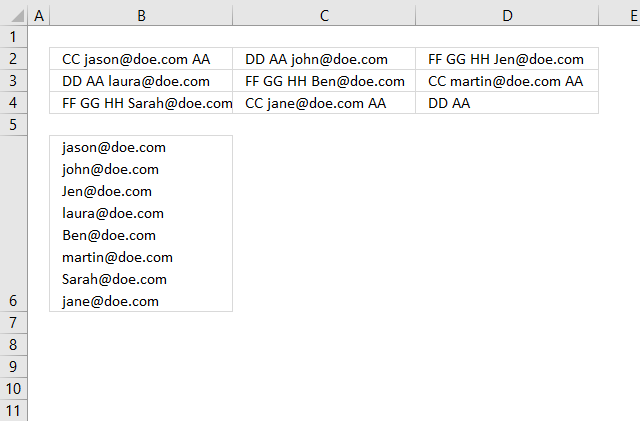It is possible to combine the array formulas in example 1 and 2, unfortunately, the formula can still only extract one email address per cell.

Array formula in cell B6:

=TEXTJOIN(CHAR(10), TRUE, IF(ISERROR(SEARCH("@", B2:D4)), "", TRIM(MID(SUBSTITUTE(TRIM(B2:D4), " ", REPT(" ", 200)), (LEN(LEFT(B2:D4, SEARCH("@", B2:D4)))-LEN(SUBSTITUTE(LEFT(B2:D4, SEARCH("@", B2:D4)), " ", "")))*200+1, 200))))

Cell B6 has "Wrap text" enabled, select cell B6 and press CTRL + 1 to open the "Format Cells" dialog box.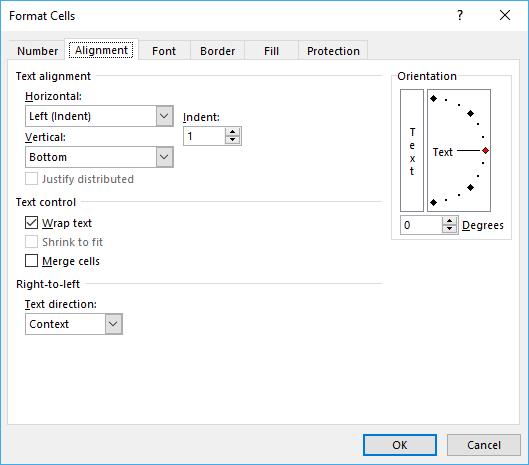## Example 4,

If you need an even better faster formula I recommend using a UDF:

Recommended articles

Filter words containing a given string in a cell range [UDF]
The image above demonstrates a User Defined Function that extracts all words containing a given string that you can specify. In […]

## Example 5,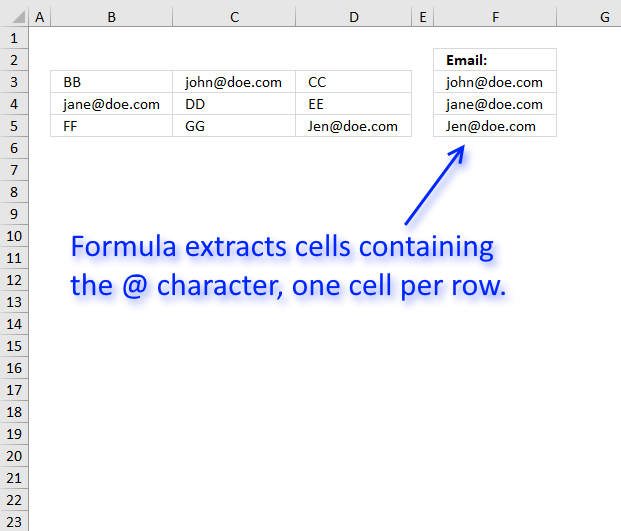The formula in cell F3 gets only one email address per row so it is very basic, however, check out the comments for more advanced formulas.

If the cell contains an email address and also other text strings it won't extract the email only, as I said, it is a very basic formula.

Array formula in F3:

=INDEX(B3:D3, 1, MIN(IF(ISERROR(SEARCH("@", B3:D3)), "", MATCH(COLUMN(B3:D3), COLUMN(B3:D3)))))

To enter an array formula, type the formula in cell F3 then press and hold CTRL + SHIFT simultaneously, now press Enter once. Release all keys.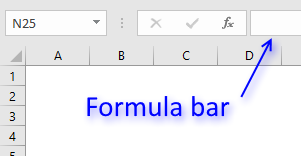The formula bar now shows the formula with a beginning and ending curly bracket telling you that you entered the formula successfully.

Don't enter the curly brackets yourself, they appear automatically.

Recommended articles

Filter words containing a given string in a cell range [UDF]
The image above demonstrates a User Defined Function that extracts all words containing a given string that you can specify. In […]

### Explaining formula in cell F3

#### Step 1 - Look for @ character in cell range

The SEARCH function allows you to find the character position of a substring in a text string, we are, however, not interested in the position only if it exists or not in the cell.

SEARCH("@", B3:D3))

becomes

SEARCH("@", {"BB","[email protected]","CC"}))

and returns {#VALUE!,5,#VALUE!}. This tells us that the second value in the array contains a @ character on position 5.

#### Step 2 - Convert array to TRUE or FALSE

The IF function can't handle error values in the logical expression so we must first convert the array to boolean values. The ISERROR function returns TRUE if the value is an error value and FALSE if not.

ISERROR(SEARCH("@", B3:D3))

becomes

ISERROR({#VALUE!,5,#VALUE!})

and returns {TRUE,FALSE,TRUE}.

#### Step 3 - Return column number if value is not an error value

IF(ISERROR(SEARCH("@", B3:D3)), "", MATCH(COLUMN(B3:D3), COLUMN(B3:D3)))

becomes

IF({TRUE,FALSE,TRUE}, "", MATCH(COLUMN(B3:D3), COLUMN(B3:D3)))

To create an array from 1 to n I use the MATCH function and COLUMN function.

IF({TRUE,FALSE,TRUE}, "", MATCH(COLUMN(B3:D3), COLUMN(B3:D3)))

becomes

IF({TRUE,FALSE,TRUE}, "", MATCH({2,3,4}, {2,3,4}))

becomes

IF({TRUE,FALSE,TRUE}, "", {1, 2,3})

and returns {"", 2,""}

#### Step 4 - Return the smallest column number

The MIN function calculates the smallest number in cell range or array.

MIN(IF(ISERROR(SEARCH("@", B3:D3)), "", MATCH(COLUMN(B3:D3), COLUMN(B3:D3))))

becomes

MIN({"", 2,""})

and returns 2.

#### Step 4 - Return value corresponding to column number

The INDEX function returns a value based on a row and/or column number.

INDEX(B3:D3, MIN(IF(ISERROR(SEARCH("@", B3:D3)), "", MATCH(COLUMN(B3:D3), COLUMN(B3:D3)))))

becomes

INDEX(B3:D3, 2)

and returns "[email protected]".

### Get Excel *.xlsx file

extract_email_2.xlsx

Learn to use regular expressions and filter emails:

Recommended articles

Extract cell references from a formula
This article demonstrates a User Defined Function that allows you to extract cell references from a formula in a given […]

Learn how to use the LIKE operator to filter emails:

Recommended articles

How to use the LIKE OPERATOR
The LIKE operator allows you to match a string to a pattern using Excel VBA. The image above demonstrates a […]